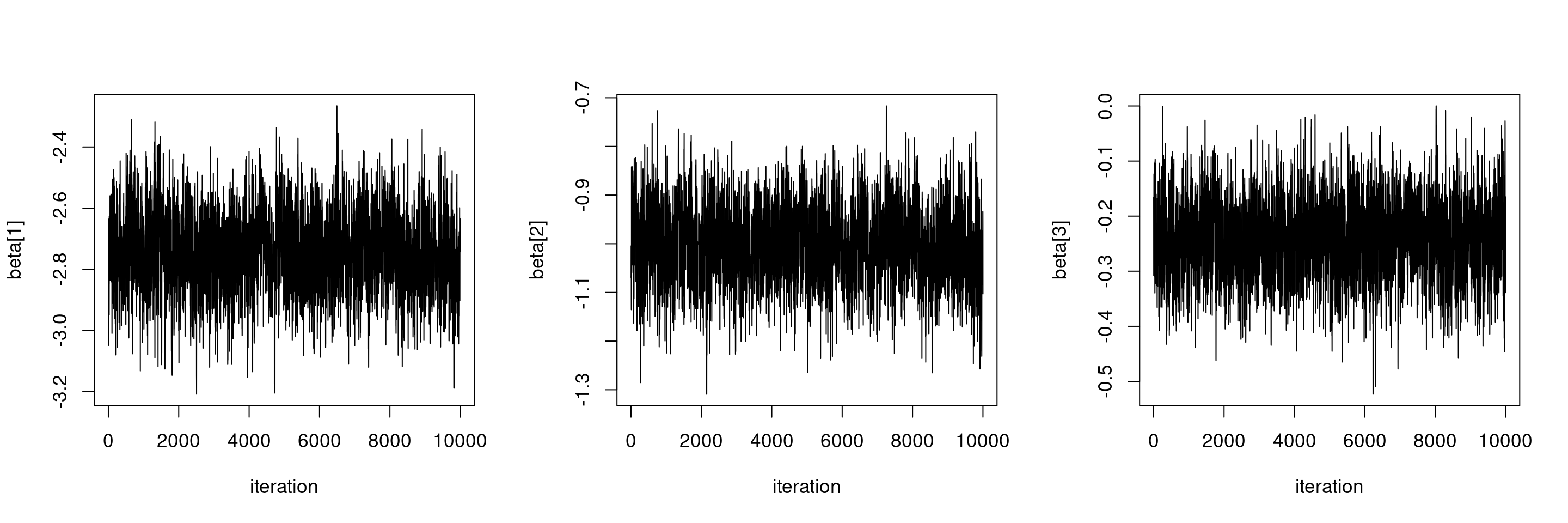## Item Response Theory

Item Response Theory (IRT) refers to a family of models that attempt to explain the relationship between latent traits and their manifestation in observations of the individual. The basic assumption is that an individual’s observable behavior is related to this latent trait. Common contexts include educational testing and health status.

In a testing context, the standard setting considers $$I$$ items and $$P$$ persons for which responses $$y_{pi} \in \{0, 1\}$$ are observed with values encoding incorrect and correct answers. The probability of a correct answer for each item/person $$\pi_{pi} = \text{Pr}(y_{pi} = 1)$$ is modeled via logistic or probit regression, conditionally on a set of parameters encoding the characteristics of respondents and items. Typically a parameter $$\eta_p$$ is defined for each respondent $$p = 1, \ldots, P$$ representing the unobserved ability of the respondent. Parameters $$\{\eta_p\}_1^P$$ are assumed to be drawn from a common distribution, taking the role of ‘random effects’.

Different models can be defined according to the number of parameters associated with each item. Here we consider two classic models for dichotomous item responses, namely the one-parameter logistic (1PL) model and the two-parameter logistic (2PL) model.

For this example, we use the Law School Admission Test (LSAT) data from the ltm package, which is a classic example in educational testing (Bock and Lieberman 1970). The dataset comprises $$1000$$ responses to $$5$$ questions.

LSATdata <- ltm::LSAT

## 1PL model

The one-parameter logistic model (1PL) assumes the presence of one parameter, $$\beta_i$$, for each item $$i = 1, \ldots, I$$ encoding the difficulty of the item. We choose a non-informative normal prior $$\mathcal{N}(0, 100)$$ for the difficulty parameters, and a uniform distribution for abilities variance.

library(nimble, warn.conflicts = FALSE)
## nimble version 1.0.1 is loaded.
##
## Note for advanced users who have written their own MCMC samplers:
##   As of version 0.13.0, NIMBLE's protocol for handling posterior
##   predictive nodes has changed in a way that could affect user-defined
##   samplers in some situations. Please see Section 15.5.1 of the User Manual.
code1PL <- nimbleCode({
for(i in 1:I) {
for(p in 1:P) {
y[p,i] ~ dbern(pi[p,i])
logit(pi[p, i]) <-  eta[p] - beta[i]
}
beta[i] ~ dnorm(0, var = 100)
}

for(p in 1:P) {
eta[p] ~ dnorm(0, sd = sd_eta)
}

sd_eta ~ dunif(0, 100)  # prior for variance components based on Gelman (2006)
})

constants <- list(I = ncol(LSATdata), P = nrow(LSATdata))
data <- list(y = LSATdata)

set.seed(1)
inits <- list(beta    = rnorm(constants$I, 0, 1), eta = rnorm(constants$P, 0, 1),
sd_eta = 3)

monitors = c("beta", "eta", "sd_eta")

model1PL <- nimbleModel(code1PL, constants, data, inits)
## Defining model
## Building model
## Setting data and initial values
## Running calculate on model
##   [Note] Any error reports that follow may simply reflect missing values in model variables.
## Checking model sizes and dimensions
cModel1PL <- compileNimble(model1PL)
## Compiling
##   [Note] This may take a minute.
##   [Note] Use 'showCompilerOutput = TRUE' to see C++ compilation details.

### Building and running an MCMC to fit the model

conf1PL <- configureMCMC(model1PL, monitors = monitors)
## ===== Monitors =====
## thin = 1: beta, eta, sd_eta
## ===== Samplers =====
## RW sampler (1006)
##   - beta[]  (5 elements)
##   - sd_eta
##   - eta[]  (1000 elements)
model1PLMCMC <- buildMCMC(conf1PL)
cModel1PLMCMC <- compileNimble(model1PLMCMC, project = model1PL)
## Compiling
##   [Note] This may take a minute.
##   [Note] Use 'showCompilerOutput = TRUE' to see C++ compilation details.

Run the model and save posterior samples.

system.time(samples1PL <- runMCMC(cModel1PLMCMC, niter = 20000, nburnin = 10000))
## running chain 1...
## |-------------|-------------|-------------|-------------|
## |-------------------------------------------------------|
##    user  system elapsed
##  26.636   0.112  26.747

### Checking the results

We can obtain a summary of the estimates of item difficulties.

betaCols <- grep("beta", colnames(samples1PL))
sd_etaCol <- grep("sd_eta", colnames(samples1PL))
samplesSummary(samples1PL[, c(betaCols, sd_etaCol)])
##               Mean     Median    St.Dev.  95%CI_low  95%CI_upp
## beta -2.7426819 -2.7411894 0.13037380 -2.9963098 -2.4855966
## beta -1.0011783 -1.0009559 0.07833695 -1.1523951 -0.8495846
## beta -0.2433821 -0.2453595 0.07151934 -0.3847355 -0.1014021
## beta -1.3106872 -1.3104853 0.08184534 -1.4721001 -1.1478006
## beta -2.1100184 -2.1103943 0.10782355 -2.3244911 -1.8996280
## sd_eta   0.7672692  0.7669722 0.06783533  0.6371932  0.9018647

Let’s check posterior convergence. In this case, the variance component appears to be the slowest mixing, and we would probably want to run the MCMC for longer.

par(mfrow = c(1, 3), cex = 1.1)
for(i in 1:5)
ts.plot(samples1PL[ , betaCols[i]], xlab = 'iteration', ylab = colnames(samples1PL)[ betaCols[i]])ts.plot(samples1PL[ , sd_etaCol], xlab = 'iteration', ylab = colnames(samples1PL)[sd_etaCol])